QUESTIONSHEET 1

(a) 750 MJ / 750,000,000 J                                                                                                                             1                           2

½ ´ 150,000 ´ 1002                                                                                                                                 1

(b) engine thrust / jet thrust                                                                                                                             1                           1

weight of plane / downward force of plane due to gravity                                                                       1

(c) 500,000 ´ d = 750 000 000                                                                                                                        1

d = 1500 m                                                                                                                               1 + 1 (units)

TOTAL  / 7

QUESTIONSHEET 2

(a) 80 N in the direction of motion / 80 N forward.                                                                                          1

(b) work done = force ´ distance                                                                                                                    1                           1

1600                                                                                                                                                        1                                       1

J                                                                                                                                                              1                                  1

(c) (i)    300 J                                                                                                                                                1                                       1

(ii)    the sledge falls / slides down the hill                                                                                                   1                         1

potential energy converted to kinetic energy.                                                                                       1                    1

TOTAL  / 7

QUESTIONSHEET 3

(a) Terminal velocity                                                                                                                                      1                             1

(b) Air resistance and gravity                                                                                                                         1                           1

(c) Air resistance reduces                                                                                                                              1                             1                                                                                                                 Gravity remains constant                           1

Gravity remains constant                                                                                                                           1

Resultant force/ unbalanced so acceleration.                                                                                              1                    1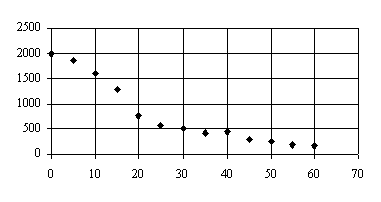(d) (i)Graph

2/3rds of paper used and correct axis                                                                                                 1                         1

Majority of correct points plotted.                                                                                                       1                         1

Anomalous result at 40secs not included in line.                                                                                  1

Smoothed line/ curve of best fit used.                                                                                                 1                         1

(ii)    Gradient calculated using straight line values between 10-15 secs                                                         1                             1

Accept answer 25-75 m/s                                                                                                                  1                           1

TOTAL  / 10

QUESTIONSHEET 4

(a) speed = distance/time                                                                                                                               1                             1

(b) 200 / 19.32                                                                                                                                               1                                  1

= 10.35m/s                                                                                                                                               1                                  1

(c) ½  ´  final speed ´  time                                                                                                                           1                           1                                                                                                                                   ½  x  7.5  x  2                                  1

½  ´ 7.5 ´ 2                                                                                                                                             1

=  7.5 m                                                                                                                                                   1                                  1

(d) speed / time                                                                                                                                             1                                  1

7.5 / 2                                                                                                                                                          1                                                                                                                                                                              1

= 3.75m/s2                                                                                                                                                                                               1                                  1

TOTAL  / 9

QUESTIONSHEET 5

(a) 2000 m                                                                                                                                                    1                                  1

(b) Alecia                                                                                                                                                      1                                       1

(c) Alecia has stopped                                                                                                                                   1                                       1

(d) 500                                                                                                                                                          1                           1          ½  x  10  x  1                                                                                                                                                       1

400

=  1.25 ms-1                                                                                                                                              1                                       1

(e) Dina overtakes Alecia                                                                                                                              1                         1

(f)      Dina                                                                                                                                                                    1                        1

(g) 2000                                                                                                                                                        1                                       1

800

= 2.5 ms-1                                                                                                                                                1

TOTAL  / 9

QUESTIONSHEET 6

(a) 1.2 s                                                                                                                                                        1                                  1

(b) deceleration= change in velocity/time                                                                                                        1                    1

= 27 / 4.2                                                                                                                                                     1                                  1

= 6.43     m/s2                                                                                                                                               1                                  1

(c) distance in 1st 1.2s = velocity ´ time                                                                                                         1                         1

= 27 ´ 1.2                                                                                                                                                1                                  1

= 32.4 m                                                                                                                                                  1                                       1

distance until car stops = (27 ´ 4.2) / 2                                                                                                       1                    1

= 56.7 m                                                                                                                                                  1                                       1

total = 32.4 + 56.7 = 89.1 m                                                                                                                      1                         1

TOTAL  / 10

QUESTIONSHEET 7

 x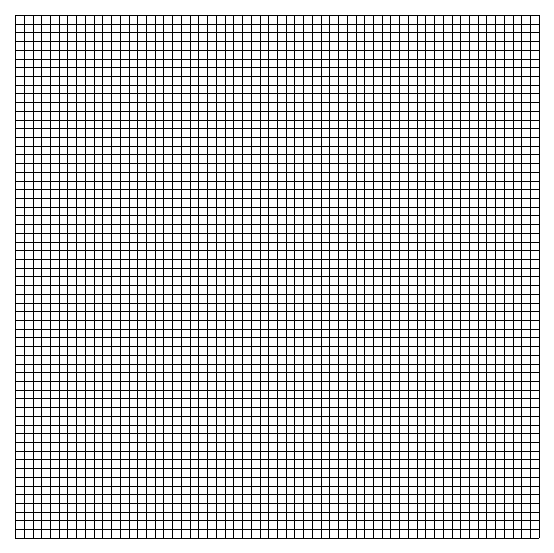(a)3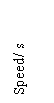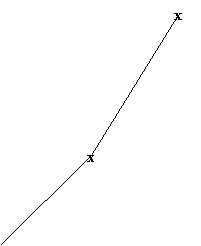1

 x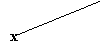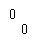20

 30

 10

 Time/ s

sensible scales                                                                                                                           1                                  1

correct plotting                                                                                                                           1                             1

correct lines drawn                                                                                                                     1                             1

(b) (i)    average acceleration = change in velocity / time                                                                                  1

= 0.5 / 5                                                                                                                                           1                                  1

= 0.1 m/s2                                                                                                                                         1                                  1

(ii)    (3 – 2) / 5                                                                                                                                         1                                  1

= 0.2 m/s2                                                                                                                                         1                                  1

(iii)   As the man pulls the lorry, he is able to accelerate more once the lorry gets moving                              1                           1

TOTAL  / 9

QUESTIONSHEET 8

(a) speed=distance/time                                                                                                                                 1                             1

(b) (i)    100 / 9.96                                                                                                                                         1                                  1

= 10.04 m/s                                                                                                                                      1                                  1

(ii)    100 / 12                                                                                                                                            1                                  1

= 8.33 m/s                                                                                                                                        1                                  1

(iii)   any viable reason:

better diet/training technique improved in 100 years/

better footwear                                                                                                                                 1                                             1

(c) (i)    100 / 40.2                                                                                                                                         1                                  1

= 2.49 m/s                                                                                                                                        1                                  1

(ii)    resistance of water slows swimmer                                                                                                    1                         1

TOTAL  / 9

QUESTIONSHEET 9

(a) constant speed/constant velocity.                                                                                                               1                         1

(b) 1 ms-2:                                                                                                                                                                      .            2

answer – 1, units – 1                                                                                                                                2

(c) region c: deceleration from 20 to 10 ms-1 in 5 sec                                                                                        1

region d: constant speed of 10 ms-1 for 20 sec                                                                                            1

region e: deceleration from 10 to 0 ms-1 in 15 sec                                                                                       1

(note: 1 mark for correct description for all 3 regions, but no quoted figures.)

(d) Bus stop, traffic jam.                                                                                                                                1                              1

TOTAL  / 7

QUESTIONSHEET 10

(a) (i)    0.9m                                                                                                                                                1                                       1

(ii)    0.3s                                                                                                                                                  1                                       1

(iii)   1.6m                                                                                                                                                1                                       1

(b) conversion of 40kg to 400N.                                                                                                                      1                           1

360 J                                                                                                                                                       1                                       1

TOTAL  / 5

QUESTIONSHEET 11

(a) 420 ´ 3 = potential energy gained

potential energy ¸ 3seconds = power = 420 W                                                                                           1                                  1

(b) Potential energy converted to kinetic energy.                                                                                              1                    1

(c) 140 ´ 3 = 420 J

working – 2, correct answer with units –1                                                                                                  3                           3                                                                                                                                                                                3

(d) 560 ´ 3 = 420 ´ time                                                                                                                                 1                    2                                                                                                                                                                  2

t = 560 ´ 3   = 4 seconds                                                                                                                           1

420

TOTAL  / 7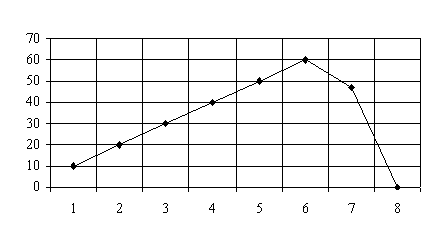QUESTIONSHEET 12

(a)    (i)

 Speed  (ms-1)

 Time (s)

correctly plotted points                                                                                                                      1                           1

line joining points.                                                                                                                              1                             1

(ii)   Rope fully stretched so speed changes from

60ms-1 to 0 / speed changes from 60ms-1 to 0.                                                                                     1

(b) (i)    formula:  deceleration = change in speed                                                                                            1                         1

time

correct answer with units: 60 ms-2                                                                                                                                        1                             1

(ii)    Upward force in the elastic                                                                                                                1                             1

(c) (i)    formula: acceleration = change in speed                                                                                             1                         1

time

correct answer with units: 10 ms-2                                                                                                                                        1                         1

(ii)    force                                                                                                                                                1                                       1

of gravity                                                                                                                                          1

TOTAL  / 10

QUESTIONSHEET 13

(a) 10 km                                                                                                                                                      1                                       1

(b) formula:  speed = distance                                                                                                                         1

time                                                                                                                                                    1

correct answer: 1 km min-1 / 60 kmh-1                                                                                                                                           1                    1

(c) (i)    car is standing still / stationary                                                                                                            1                         1

(ii)    formula: speed = distance                                                                                                                  1

time                                                                                                                                              1

correct answer: 1.5 km min-1 / 90 km h-1                                                                                                                           1                    1

(d) use of re-arranged formula: time = distance                                                                                                1

speed                                                                                                             1

correct calculation of time for BC: 6 min                                                                                                    1                    1

correct final answer: 12 min                                                                                                                      1                           1

TOTAL  / 9

QUESTIONSHEET 14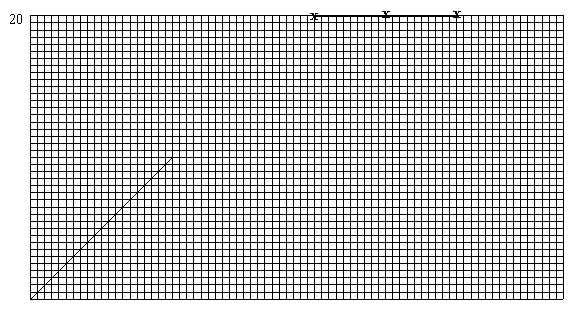(a)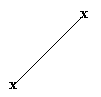15

 10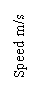x

 5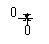30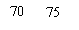10

 20

 40

 50

 60

 Time/ s

sensible scales                                                                                                                           1                                  1

correct plotting                                                                                                                           1                             1

correct lines drawn                                                                                                                     1                             1

(b) ½  ´  final velocity  ´  time                                                                                                                        1                           1

½  ´  15  ´  30                                                                                                                                         1                                  1

=  225 m                                                                                                                                                  1                                  1

(c) moving at constant speed                                                                                                                          1                           1

(d) (i)    line drawn from end of graph to ´ axis at 75 s                                                                                     1

(ii)    change in velocity / time                                                                                                                    1                           1

=  20 / 15                                                                                                                                          1                                  1

=  1.33 m/s2                                                                                                                                                                                  1                                  1

TOTAL  / 11

QUESTIONSHEET 15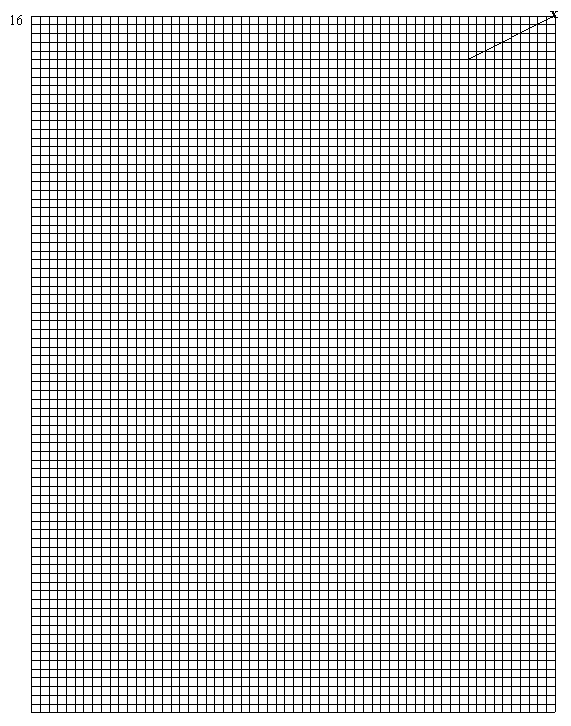(a)     (i)

 x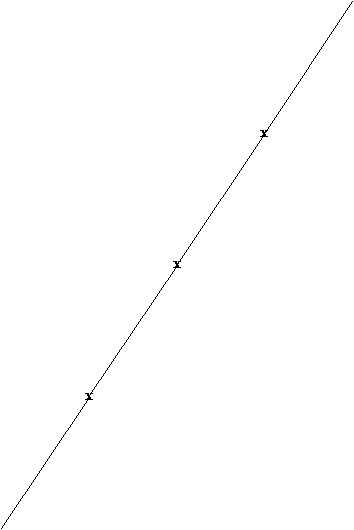14

 122

 1010

 8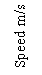6

 4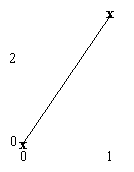2

 3

 4

 5

 6

 Time/ s

sensible scales                                                                                                                           1                                  1

correct plotting                                                                                                                           1                             1

correct lines drawn                                                                                                                     1                             1

(Continued...)

QUESTIONSHEET 15 CONTINUED

(ii)    speed/time                                                                                                                                        1                                  1

= 15/5                                                                                                                                              1                                       1

= 3m/s2                                                                                                                                                                                                 1                                  1

(iii)    ½  ´  final speed ´  time                                                                                                                   1                           1                                                                                                                                    ½  x  15  x  5                                  1

½  ´ 15 ´ 5                                                                                                                                     1

=  37.5 m                                                                                                                                        1                                  1

(b) (i)    line parallel to time axis                                                                                                                     1                           1

(ii)    anywhere on last line sloping down                                                                                                     1                         1

TOTAL  / 11

QUESTIONSHEET 16

(a) speed=distance/time                                                                                                                                 1                             1

=15 / 0.6                                                                                                                                                  1                                  1

=25m/s                                                                                                                                                    1                                  1

(b) 63 –15 = 48m                                                                                                                                          1                             1

(c) none                                                                                                                                                            1                                                                                                                                                                              1

(d) Two from

mass of car, friction surfaces e.g. brakes,

TOTAL  / 7

QUESTIONSHEET 17

(a)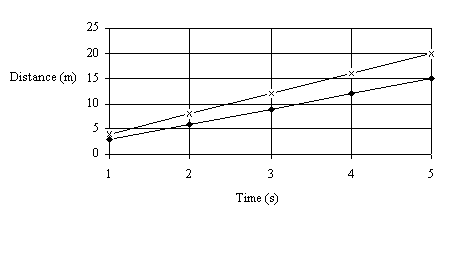Deduct one mark for each incorrectly plotted point                                                                                     3

(b) (i)    Kris: 4 ms-1                                                                                                                                                                                   1                                  1

Joshua: 3 ms-1                                                                                                                                                                              1                                  1

average speed = distance                                                                                                                1

time                                                                                                                                             1

(ii)    Kris’s car                                                                                                                                         1                                  1

(c) kinetic energy to heat and sound.                                                                                                               1                         1

TOTAL  / 8

QUESTIONSHEET 18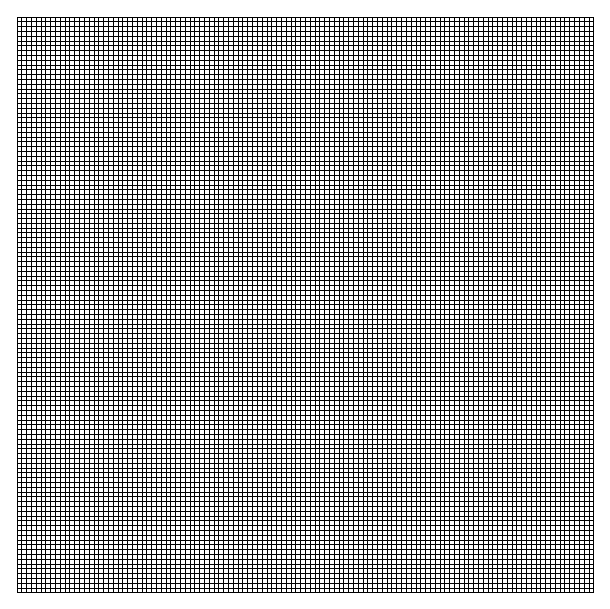(a)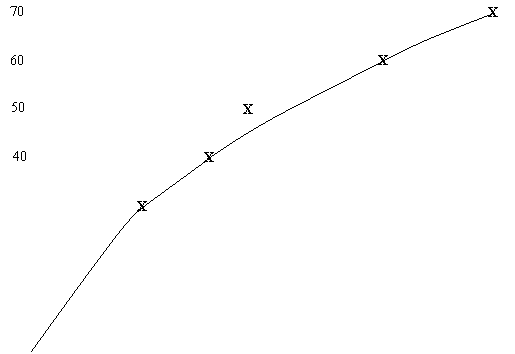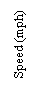30

 20

 10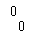100

 90

 10

 30

 40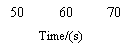80

 20

sensible scales                                                                                                                           1                                  1

correct plotting                                                                                                                           1                             1

correct lines drawn                                                                                                                     1                             1

(b) 50 mph                                                                                                                                                    1                                  1

(c) (i)      distance travelled while reacting                                                                                                      1                         1

(ii)     distance travelled while braking                                                                                                        1                         1

(d) Two from

wet roads, driver may have drunk alcohol, driver tired,

bald tyres                                                                                                                                                 2                                  2

TOTAL  / 8

QUESTIONSHEET 19

(a) Gravity/ weight                                                                                                                                         1                             1

(b) stays the same.                                                                                                                                        1                             1

(i)    Unbalanced                                                                                                                                      1                                  1

(ii)    Unbalanced                                                                                                                                      1                                   1

(iii)   Balanced                                                                                                                                          1                                  1

(d) Constant/ steady/ same speed                                                                                                                   1                           1

(e) Stage 1:  Acceleration                                                                                                                               1                           1                                                                                                             Resultant force is downward                           1

Resultant force is downward                                                                                                       1

Stage 2:  Acceleration                                                                                                                               1                           1

Resultant force is upward.                                                                                                          1                           1

TOTAL  / 10

QUESTIONSHEET 20

(a) 40m/s                                                                                                                                                      1                                       1

(b) (i)    train travelling at 40m/s (constant speed)                                                                                            1                    1

(ii)    train decelerates                                                                                                                               1                                  1

(c) B                                                                                                                                                            1                                       1

(d) distance = speed ´ time                                                                                                                            1                           1

time = 200 – 50 s                                                                                                                                      1                             1

distance = 40 ´ 150                                                                                                                                  1                             1

= 600 m                                                                                                                                                       1                                  1

TOTAL  / 8